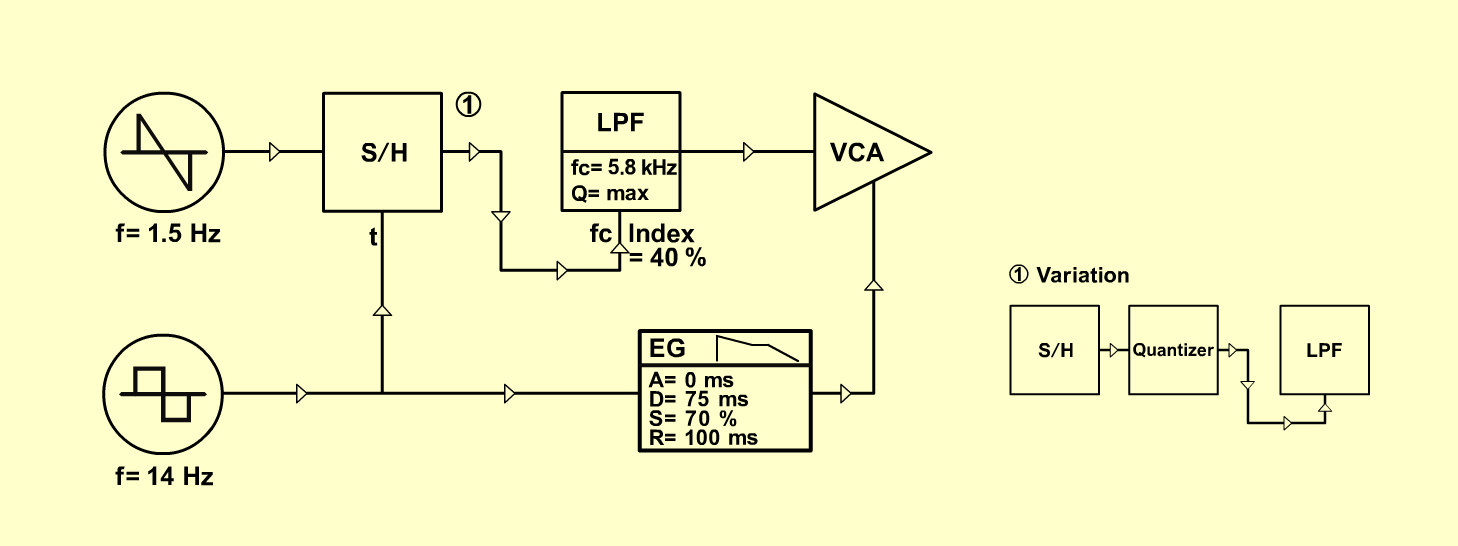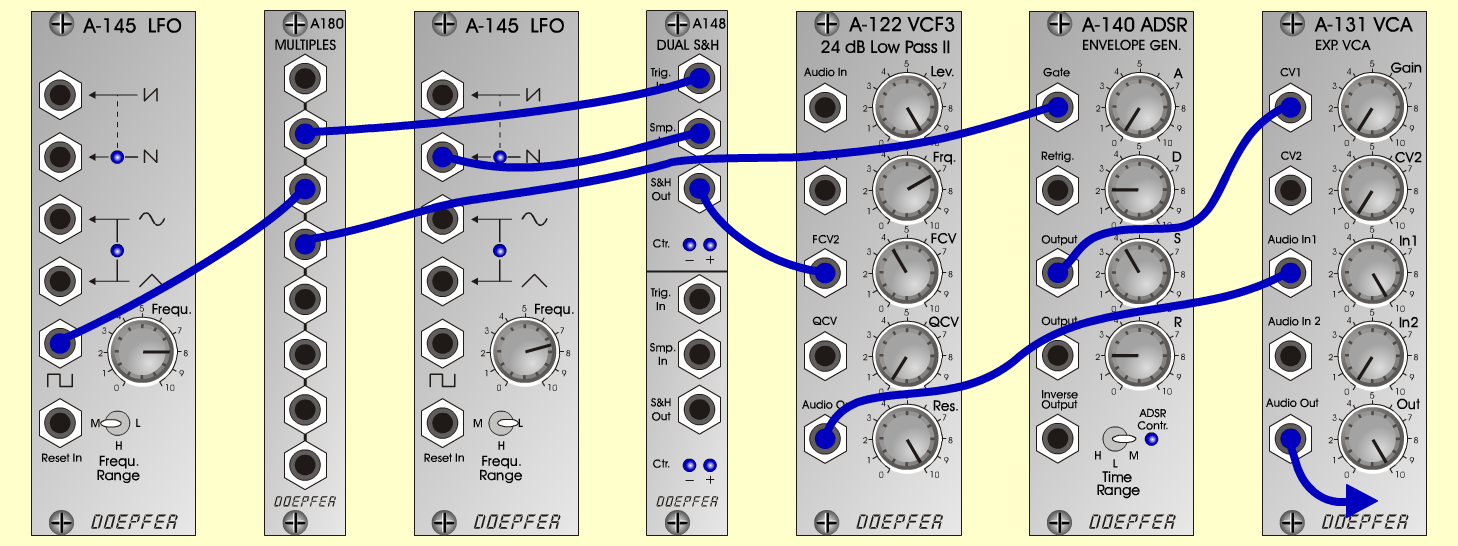JumperFXD o e p f e r A - 100 Connections: Settings: A-122 (Audio Out)  <=>  A-131 (Audio In) A-180  <=>  A-148 (Trig In) A-180  <=>  A-140 (Gate) A-140 (Output)  <=>  A-131 (CV1) A-145/2 (Saw Up)  <=>  A-148 (Smp In) A-148 (S&H Out)  <=>  A-122 (FCV 2) Trigger and Gate: Seq or A-145/1 (Pulse)  <=>  A-180 A-122 (Level = 10, Freq = 7, FCV  = 4, Res = 10) A-140 (A = 0, D = 2, S = 4, R = 2, Range = M) A-131 (Gain = 0, Audio In 1 = 10,  Audio Out = 10) A-145/1 (Frq = 8, Range = M) A-145/2 (Frq = 7.5, Range = L) Notes: Variation: A-148 (S&H Out)  <=>  A-156 (Q2 CV In) A-156 (Q2 CV Out)  <=>  A-122 (FCV 2) Axel Jungkunst, March 2001Sound samples Jumper Jumper (reverb)